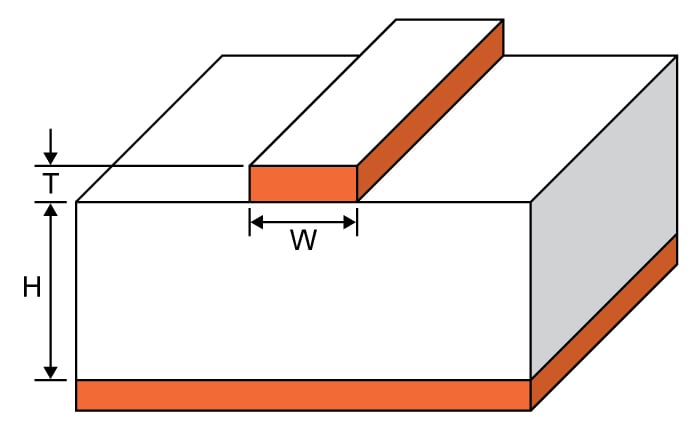# Microstrip Wavelength Calculator

## A tool designed to calculate the guided wavelength inside a microstrip.

mm

### Overview

A microstrip is a transmission line used for signals in the microwave range. It was invented because of the undesirable effects on coaxial cable when signals in the microwave range propagate through it. The wavelength of the signal that can propagate effectively inside a microstrip is called the guided wavelength. This calculator will help you calculate the guided wavelength, provided the dimensions of the microstrip are given as well as the velocity of propagation, the microstrip material's dielectric constant, and the input frequency.To use the calculator, place values in either the velocity of propagation field or the dielectric constant field. Then enter the values of the width, height, and the input frequency. The velocity of propagation is calculated using the formulas presented below.

### Equations

$$\lambda_{g} = \frac{300}{f_{GHz}\sqrt{\epsilon_{eff}}}$$

Where:

$$\epsilon_{eff} = \frac{\epsilon_{R} + 1}{2} + \frac{\epsilon_{R} - 1}{2}\left\{\frac{1}{\sqrt{1+12(\frac{H}{W})}}+0.04\left[1-\left(\frac{W}{H}\right)^{2}\right]\right\}$$      if $$\frac{W}{H} < 1$$

$$\epsilon_{eff} = \frac{\epsilon_{R}+1}{2}+\left[\frac{\epsilon_{R}-1}{2\sqrt{1+12(\frac{H}{W})}}\right]$$   if $$\frac{W}{H} > 1$$

### Applications

A microstrip is constructed using a conductor and a wide ground plane separated by a dielectric layer. Microstrips have become widely popular in microwave circuits not only because of their inherent characteristics, but also because of the convenience they provide. Microstrips are created simply by etching paths on printed circuit boards, which allow for a low production cost -- another reason for their popularity.

You might notice that the equation for solving the guided wavelength of a microstrip involves the "effective" dielectric constant. Note that this is not the dielectric constant of the material between the conductor and the ground plane (known as the substrate). This is because part of the fields from the microstrip conductor exists in air. Thus, there is a need to calculate the effective dielectric constant which is less than the substrate's dielectric constant.

The effective dielectric constant can be calculated using the formulas above, but the formula to be used depends on the the ratio of the width to the height of the microstrip line (W/H), as well as the dielectric constant of the substrate material.

Once the effective dielectric constant is calculated, the guided wavelength through the microstrip can be calculated by dividing the free space wavelength (which is the wavelength of light) by the square root of the effective dielectric constant.